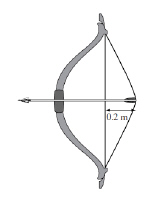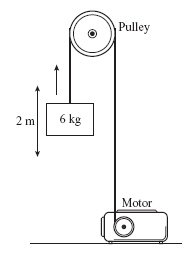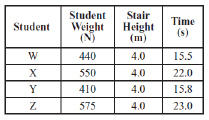Name:    MCAS Work and Power 2004-2014

Multiple Choice
Identify the choice that best completes the statement or answers the question.

1.

An archer pulls back the bowstring to prepare to shoot an arrow as shown below.She uses an average force of 40 N, moving the bowstring 0.2 m. How much energy is stored in the bow? (MCAS 2004)
 a. 8 J c. 24 J b. 16 J d. 36 J

2.

At a weightlifting competition, two competitors lifted the same weight to the same height. The second competitor accomplished the lift 2 seconds faster than the first competitor. This demonstrated that the second competitor had more ____. (MCAS 2004)
 a. energy than the first c. power than the first b. inertia than the first d. work than the first

3.

A man ran on a treadmill for 1,800 seconds. At the end of his run, the treadmill indicated his energy output as 240,000 J. What average power did he generate? (MCAS 2005)
 a. 666 W c. 66 W b. 133 W d. 7 W

4.

Two students each carry a box up to the third floor of a building. The total mass of each student and the box she is carrying is the same. Roberta makes the trip in 45 s while Mary takes 30 s. Which of the following statements best describes this task? (MCAS 2006)
 a. Roberta does more total work than Mary. b. Mary does more total work than Roberta. c. Roberta has a greater power output than Mary. d. Mary has a greater power output than Roberta.

5.

The diagram shows a motor being used to lift a load with the use of a pulley. (MCAS 2006)The motor is lifting the 6 kg box at a constant velocity. How much work is done on the box to lift it 2 m? (Assume no resistance from the pulley.)
 a. 3 J b. 4 J c. 60 J d. 120 J

6.

The motor of one car is more powerful than the motor of another car. Which of the following must be true of the more powerful motor? (MCAS 2007)
 a. It can do work more quickly. c. It can burn fuel more efficiently. b. It can operate for a longer time. d. It can store more potential energy.

7.

A crane lifts two 100 kg loads of building materials to the top of a building under construction. The first load is lifted in 10 s and the second load is lifted in 13 s. Which of the following statements best compares the work and power expended by the crane lifting the two loads? (MCAS 2007)
 a. The crane does more work and expends more power for the first lift. b. The crane does the same work and expends the same power for each lift. c. The crane does the same work for each lift but expends more power for the first lift. d. The crane does more work in the second lift but expends the same power for each lift.

8.

How much power is required to carry a 35 N package a vertical distance of 18 m if the work on the package is accomplished in 30 s? (MCAS 2008)
 a. 16 W c. 58 W b. 21 W d. 630 W

9.

Which of the following is the best example of work being done on an object? (MCAS 2009)
 a. holding a 50 kg barbell c. keeping a board in place b. lifting a bag of groceries d. pushing on a car that will not move

10.

Four students of different weights timed themselves walking up a set of stairs. Their data are shown below.Which student used the most power while walking up the stairs? (MCAS 2009)
 a. student W c. student Y b. student X d. student Z

11.

A man applies a force of 100 N to a rock for 60 seconds, but the rock does not move. What is the amount of work done by the man on the rock? (MCAS 2009)
 a. 0.0 J c. 100 J b. 6.0 J d. 6000 J

12.

In a competition, weightlifter 1 lifts a 100 kg weight from the floor. Weightlifter 2 also lifts a 100 kg weight to the same height above the floor, but takes a longer time to do so.
Which of the following statements describes the work done and the power used by the weightlifters? (MCAS 2010)
 a. Weightlifter 2 does the same work and uses less power compared with weightlifter 1. b. Weightlifter 2 does less work and uses the same power compared with weightlifter 1. c. Weightlifter 2 does the same work and uses more power compared with weightlifter 1. d. Weightlifter 2 does more work and uses the same power compared with weightlifter 1.

13.

A person is using a force of 200 N to push a box. How much power does the person need to push the box a distance of 4 m in 10 s? (MCAS 2010)
 a. 40 W c. 200 W b. 80 W d. 500 W

14.

If 105 J of energy is used in 103 s, what is the average power consumption in watts? (MCAS 2011)
 a. 102 W c. 1011 W b. 108 W d. 1015 W

15.

A rock climber weighs 550 N and is carrying a backpack that weighs 50 N. How much total work has been done after she climbs 12 m? (MCAS 2011)
 a. 50 J c. 6000 J b. 600 J d. 7200 J

16.

A 1000 kg automobile is traveling at an initial speed of 20 m/s. It is brought to a complete stop in 5 s over a distance of 50 m. What is the work done in stopping the automobile? (MCAS 2012)
 a. 10,000 J c. 50,000 J b. 40,000 J d. 200,000 J

17.

An electric fan has a power output of 60 W. How much work is done if the fan operates for 120 s? (MCAS 2013)
 a. 0.5 J c. 120 J b. 60 J d. 7200 J

18.

An athlete is training for a race by performing timed trials of sprints up a staircase. Which set of variables most directly affects the athlete’s power? (MCAS 2013)
 a. body weight, height climbed, width of steps b. body weight, time spent climbing, width of steps c. height climbed, time spent climbing, body weight d. height climbed, time spent climbing, width of steps

19.

A book weighing 18.0 N is lifted from the floor and placed on a shelf 1.5 m high. What is the minimum amount of work required to accomplish this task? (MCAS 2014)
 a. 12 J c. 120 J b. 27 J d. 270 J

Essay

20.

Two athletes are lifting weights. Athlete X lifts 445 N a distance of 2 m in 4 s. Athlete Y lifts 445 N a distance of 2 m in 1 s. Assume that each athlete lifts the weights over his head the same distance from the floor. (MCAS 2009)
a.       Calculate the amount of work each athlete does. Show your calculations and include units in your answer.
b.       Compare the amounts of work done by the two athletes. Explain your answer.
c.       Calculate the power each athlete uses. Show your calculations and include units in your answer.
d.       Compare the power used by athlete X with the power used by athlete Y. Explain your answer.

21.

A student lifts a 10.0 kg box from the floor at a constant speed to a shelf that is 1.5 m above the floor. (MCAS 2012)

a.       Construct a free-body force diagram that represents the forces acting on the box when it is 1.0 m above the floor. Be sure to label your diagram. (* See Ch. 5 - Forces in Equilibrium)
b.       Explain how you know that work is being done on the box, and how the work results in a change in the energy of the box.
c.       Calculate the amount of work done on the box and the change in potential energy of the box. Show your calculations and include units in your answer.

22.

A 50 kg student climbs 3 m to the top of a set of stairs. (MCAS 2014)

a.       Calculate the change in the student’s gravitational potential energy from the bottom to the top of the stairs. Show your calculations and include units in your answer.
b.       How much total work does the student do while climbing the stairs? Show your calculations or explain your reasoning. Include units in your answer.

It takes the student 30 s to climb to the top of the stairs.

c.       What is the average power generated by the student climbing the stairs? Show your calculations and include units in your answer.

The next day the student carries a 10 kg backpack up the same stairs and again takes 30 s to reach the top of the stairs.

d.       Is the average power you calculated in part (c) greater than, less than, or equal to the average power the student generated the next day? Explain your answer.